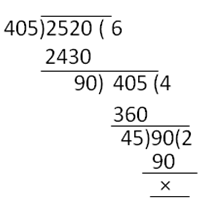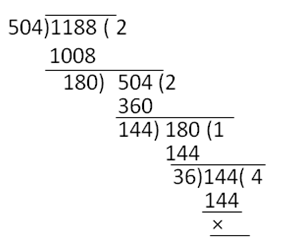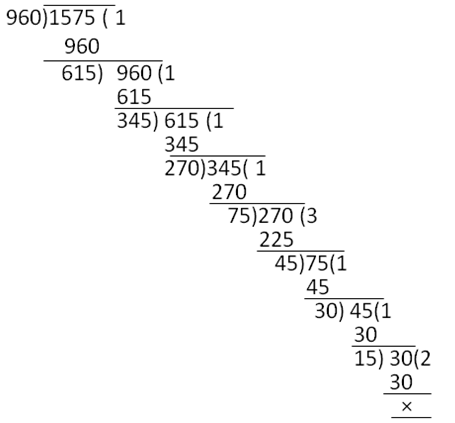RS Aggarwal Solutions: Real Numbers - 1

# RS Aggarwal Solutions: Real Numbers - 1 - Mathematics (Maths) Class 10

Q.1. What do you mean by Euclid's division lemma?

Solution: For any two given positive integers a and b there exist unique whole numbers q and r such that

a = bq + r, when 0 ≤ r < b.
Here, we call 'a' as dividend, b as divisor, q as quotient and r as remainder.
Dividend = (Divisor × Quotient) + Remainder

Q.2. A number when divided by 61 gives 27 as quotient and 32 as remainder. Find the number.
Solution: Using Euclid’s divison Lemma
Dividend = (Divisor × Quotient) + Remainder
= (61 × 27) + 32
= 1647 + 32
= 1679
Required number = 1679

Q.3. By what number should 1365 be divided to get 31 as quotient and 32 as remainder?
Solution: By Euclid's Division Algorithm, we have:
Dividend = (Divisor X Quotient) + Remainder
1365 = (Divisor × 31) + 32
(1365 - 32) / 31 = Divisor
⇒ 1333 / 31 = Divisor
Therefore, Divisor = 43

Q.4. Using Euclid's algorithm, find the HCF of:
(i) 405 and 2520
(ii) 504 and 1188
(iii) 960 and 1575
Solution:
(i) On dividing 2520 by 405, we get
Quotient = 6, remainder = 90
Therefore, 2520 = (405 × 6) + 90

Dividing 405 by 90, we get

Quotient = 4,

Remainder = 45

Therefore, 405 = 90 × 4 + 45
Dividing 90 by 45

Quotient = 2, remainder = 0
Therefore, 90 = 45 × 2
H.C.F. of 405 and 2520 is 45(ii) Dividing 1188 by 504, we get

Quotient = 2, remainder = 180
Therefore, 1188 = 504 × 2 + 180

Dividing 504 by 180

Quotient = 2, remainder = 144

Therefore, 504 = 180 × 2 + 144

Dividing 180 by 144, we get

Quotient = 1, remainder = 36

Dividing 144 by 36

Quotient = 4, remainder = 0

Therefore, H.C.F. of 1188 and 504 is 36(iii) Dividing 1575 by 960, we get
Quotient = 1, remainder = 615
1575 = 960 x 1 + 615
Dividing 960 by 615, we get Quotient = 1, remainder = 345
960 = 615 x 1 + 345
Dividing 615 by 345 Quotient = 1, remainder = 270
615 = 345 x 1 + 270
Dividing 345 by 270, we get Quotient = 1, remainder = 75
345 = 270 x 1 + 75
Dividing 270 by 75, we get Quotient = 3, remainder =45
270 = 75 x 3 + 45
Dividing 75 by 45, we get Quotient = 1, remainder = 30
75 = 45 x 1 + 30
Dividing 45 by 30, we get Remainder = 15, quotient = 1
45 = 30 x 1 + 15
Dividing 30 by 15, we get Quotient = 2, remainder = 0
H.C.F. of 1575 and 960 is 15Q.5. Show that every positive integer is either even or odd.
Solution: Let a be a given positive integer.
On dividing a by 2, let q be the quotient and r be the remainder.
Then, by Eudid's algorithm, we have
a = 2q+ r, where 0 < r < 2
⇒ a = 2q + r, where r = 0,1
⇒ a = 2q or a = 2q + 1
When a = 2q for some integer q, then clearly a is even.

When a = 2q + 1 for some integer q, then dearly a is odd.

Thus, every positive integer is either even or odd.

Q.6. Show that any positive odd integer is of the form (6m + 1) or (6m + 3) or (6m + 5), where m is some integer.
Solution: Let a be a given positive integer.
On dividing a by 6, let q be the quotient and r be the remainder.
Then, by Eudid's algorithm, we have
a = 6q + r, where 0 < r < 6
⇒ a = 6q + r, where r = 0,1,2,3,4,5,6
⇒ a = 6q or a = 6q + 1 or a = 6q + 2 or a = 6q + 3 or a = 6q + 4 or a = 6q + 5
But, a = 6q, a = 6q + 2, a = 6q + 4 gives even values of a.
Thus, when a is odd, it is of the form
6q + 1, 6q + 3 or 6q + 5 for some integer q.

Q.7. Show that any positive odd integer is of the form (4m + 1) or (4m + 3), where in is some integer.
Solution:
Let a be a given positive odd integer.
On dividing aby 4, let qbe the quotient andr be theremainder.
Then, by Eudid's algorithm, we have
a = 4m + r, where 0 < r < 4
⇒ a = 4m + r, where r = 0,1,2,3
⇒ a = 4m or a = 4m + 1 or a = 4m + 2 or a = 4m + 3
But, a = 4m and a = 4m + 2 = 2(2m + 1) are clearly even.
Thus, when ais odd, it is of the form a = (4m + 1)or (4m + 3) for some integer m.

The document RS Aggarwal Solutions: Real Numbers - 1 | Mathematics (Maths) Class 10 is a part of the Class 10 Course Mathematics (Maths) Class 10.
All you need of Class 10 at this link: Class 10

## Mathematics (Maths) Class 10

115 videos|478 docs|129 tests

## FAQs on RS Aggarwal Solutions: Real Numbers - 1 - Mathematics (Maths) Class 10

 1. What are real numbers?Ans. Real numbers are a set of numbers that include both rational and irrational numbers. They can be represented on a number line and can be positive, negative, or zero.
 2. How are real numbers different from rational numbers?Ans. Real numbers include both rational and irrational numbers, while rational numbers are those that can be expressed as a fraction. Real numbers are a broader category that includes all possible numbers on the number line.
 3. What are the properties of real numbers?Ans. Real numbers have several properties, including closure under addition, subtraction, multiplication, and division. They also follow the associative, commutative, and distributive laws.
 4. Can real numbers be negative?Ans. Yes, real numbers can be negative. They can be any number on the number line, including negative numbers, positive numbers, and zero.
 5. What is the significance of real numbers in mathematics?Ans. Real numbers are fundamental in mathematics as they form the basis for various mathematical operations and calculations. They are used in various branches of mathematics, such as algebra, calculus, and geometry.

## Mathematics (Maths) Class 10

115 videos|478 docs|129 testsExplore Courses for Class 10 examSignup to see your scores go up within 7 days! Learn & Practice with 1000+ FREE Notes, Videos & Tests.
10M+ students study on EduRev
Track your progress, build streaks, highlight & save important lessons and more!
Related Searches

,

,

,

,

,

,

,

,

,

,

,

,

,

,

,

,

,

,

,

,

,

;